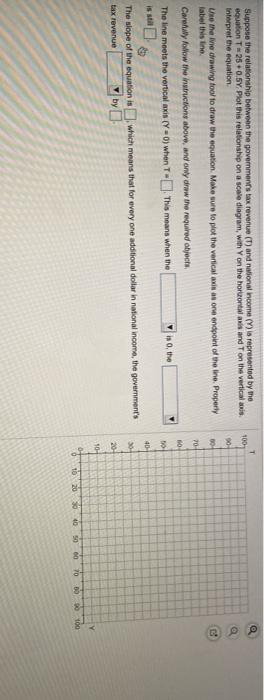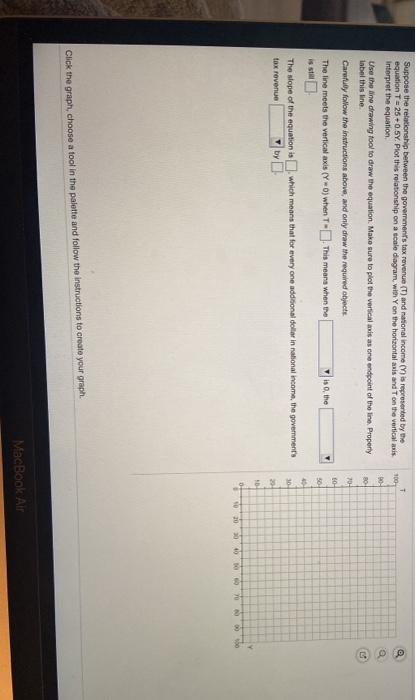### Create an Account

Already have account?

### Forgot Your Password ?

Home / Questions / Suppose the relationship between the government's tax revenue (1) and national income (V) ...

# Suppose the relationship between the government's tax revenue (1) and national income (V) in represented by me equation T250.5Y Plot this relationship on a scale diagram, with Yon the horontal and

Suppose the relationship between the government's tax revenue (1) and national income (V) in represented by me equation T250.5Y Plot this relationship on a scale diagram, with Yon the horontal and Ton the verticals Interpret the equation Une the line drawing tool to draw the equation Make sure to plot the verticale as one endpoint of the ine. Property label this line Carey follow the instructions above, and only draw the required objects, The line meets the vertical axis (y=0) when - This means when the iso, the 70 BO 50 40 which means that for every one additional dollar in national income, the government's The slope of the equation is tax revenue by 20 10- y 10 20 30 40 00 70 30100
T 100 30 M 70- Suppose the relationship between the government's tax revenue (T) and national Income (Y) is represented by the equation T25 +0.5Y. Plot this relationship on a scale diagram, with Yon the horizontal axis and on the vertical axis Interpret the equation Use the line drawing tool to draw the equation. Make sure to plot the verticals as one endpoint of the line. Property label this line Carnaby follow the instructions above, and only draw the required objecte The line meets the vertical axis (Y) when T- This means when the is the T is to The slope of the equation is which means that for everyone additional dollar in national income, the government's tox revenue 0 30 by 0 10 30 20 yo 40 Click the graph, choose a tool in the palette and follow the instructions to create your graph. MacBook AirMay 07 2021 View more View Less

#### Answer (Solved)Subscribe To Get Solution